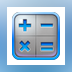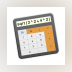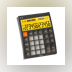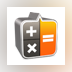Thank you for using our Mac software portal. Sorry, but Percentages Calculator for Mac does not have a direct download. Use the link below and download the required product from the App Store.

•Calculator PRO Calculator Expert is the most precise mathematical app for your Mac. It lets... \$4.99 DOWNLOAD
•Calculator + ƒ ◆ Calculator + ƒ is a powerful, extensible calculator accepting natural formula... \$4.99 DOWNLOAD
•Calculator + Calculator + is an advanced, extensible calculator accepting natural (infix)... \$5.99 DOWNLOAD
•Calculator LCD Calculator LCD — fast and convenient calculator that is always at hand.... \$0.99 DOWNLOAD
•Calculator LCD Mini Calculator LCD Mini is a compact and simple calculator that is always at hand.... DOWNLOAD
Related PC softwarePercentage Calc

Calculators